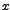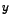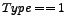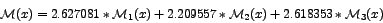DATA MINING
Desktop Survival Guide
by Graham Williams## Step by Step

The learner deployed in the AdaBoost algorithm is typically a decision tree learner that builds no more than a single split decision tree (also called a decision stump). Such a decision tree can be built in R using rpart and we illustrate this in the following code segments.

First we load the wine dataset and extract the input variables () and the output variable (). For a simple application of the algorithm, we'll have only a binary output (predicting), and again for mathematical convenience we'll predict 1 or -1:

 > library(rpart) > load("wine.RData") > N <- nrow(wine) # 178 > M <- ncol(wine) # 14 > x <- as.matrix(wine[,2:M]) > y <- as.integer(wine[,1]) > y[y>1] <- -1 > y  1 1 1 1 1 1 1 1 1 1 1 1 1 1 1 1 1 1 1 1 1 1 1 1 1 [...]  -1 -1 -1 

Now we'll initialise the entity weights and build the first model. We first set the weights to all be the same, and then set up an rpart.control structure for building a decision tree stump. The control simply includes the maxdepth option set to 1 so that a single level tree is built:

 > w <- rep(1/N, N) > w  0.005617978 0.005617978 0.005617978 0.005617978 0.005617978 0.005617978 [...]  0.005617978 0.005617978 0.005617978 0.005617978 > control <- rpart.control(maxdepth=1) > M1 <- rpart(y ~ x, weights=w/mean(w), control=control, method="class") > M1 n= 178 node), split, n, loss, yval, (yprob) * denotes terminal node 1) root 178 59 -1 (0.66853933 0.33146067) 2) x.Proline< 755 111 2 -1 (0.98198198 0.01801802) * 3) x.Proline>=755 67 10 1 (0.14925373 0.85074627) * 

We see that the decision tree algorithm has chosen Proline to split the data on, at a split point of 755. For Proline less than 755 the decision is -1 with probability 0.98, and for Proline greater than or equal to 755 the decision is 1 with probability 0.85.

We now need to find those entities which are incorrectly classified by the model. The R code here calls predict to apply the model M1 to the dataset it was built from. From this result we get the second column which is the list of probabilities for each entity being in class 1. If this probability is above 0.5 then the result is 1, otherwise it is -1 (multiplying the logical value by 2 and then subtracting 1 achieves this since TRUE is regarded as 1 and FALSE as 0). The resulting class is then compared to the y's and which returns the index of those entities for which the prediction differs from the actual class.

 > ms <- which(((predict(M1)[,2]>0.5)*2)-1 != y) > names(ms) <- NULL > ms  5 44 71 74 75 96 142 145 146 158 176 177 

We can now calculate the model weight and update the entity weights, dividing by the resulting sum of weights to get a normalised value (so that sum(w) is 1:

 > e1 <- sum(w[ms])/sum(w) # 0.06741573 > a1 <- log((1-e1)/e1) # 2.627081 > w[ms] <- w[ms]*exp(a1) > w[ms]  0.07771536 0.07771536 0.07771536 0.07771536 0.07771536 0.07771536  0.07771536 0.07771536 0.07771536 0.07771536 0.07771536 0.07771536 

We build our second model:

 > M2 <- rpart(y ~ x, weights=w/mean(w), control=control, method="class") > M2 n= 178 node), split, n, loss, yval, (yprob) * denotes terminal node 1) root 178 45.3935700 -1 (0.744979920 0.255020080) 2) x.Flavanoids< 2.31 101 0.5361446 -1 (0.995381062 0.004618938) * 3) x.Flavanoids>=2.31 77 17.0672700 1 (0.275613276 0.724386724) * > ms <- which(((predict(M2)[,2]>0.5)*2)-1 != y) > names(ms) <- NULL > ms  28 64 66 67 72 74 80 82 98 99 100 110 111 121 122 124 125 126 127  129 > e2 <- sum(w[ms])/sum(w) # 0.09889558 > a2 <- log((1-e2)/e2) # 2.209557 > w[ms] <- w[ms]*exp(a2) > w[ms]  0.05118919 0.05118919 0.05118919 0.05118919 0.05118919 0.70811707  0.05118919 0.05118919 0.05118919 0.05118919 0.05118919 0.05118919  0.05118919 0.05118919 0.05118919 0.05118919 0.05118919 0.05118919  0.05118919 0.05118919 

And then our third model:

 > M3 <- rpart(y ~ x, weights=w/mean(w), control=control, method="class") > M3 n= 178 node), split, n, loss, yval, (yprob) * denotes terminal node 1) root 178 27.60091 -1 (0.84493870 0.15506130) 2) x.Proline< 987.5 134 12.09805 -1 (0.92554915 0.07445085) * 3) x.Proline>=987.5 44 0.00000 1 (0.00000000 1.00000000) * > ms <- which(((predict(M3)[,2]>0.5)*2)-1 != y) > names(ms) <- NULL > ms  5 20 21 22 25 26 29 36 37 40 41 44 45 48 57 > e3 <- sum(w[ms])/sum(w) > e3  0.06796657 > a3 <- log((1-e3)/e3) > a3  2.618353 

The final model, if we chose to stop here, is then: